# Number of replications

BioVinci parses and handles any matrix as columns. This feature is designated for simple drag-and-drop manipulation, but the data has to be formatted in a specific way. In the data-uploading window, there is a box allowing users to provide the number of replications in their data. What is the actual meaning of this number?

### Transformation of input data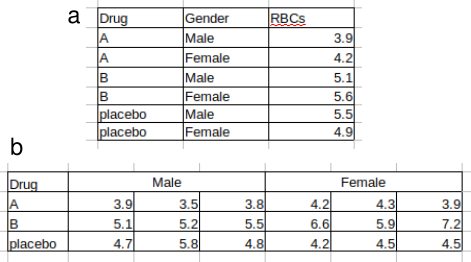Figure 2. Common data formats supported by the platform. The data shows the red blood cell count (RBCs) controlled by two factors: drug and gender. (a) There is no replication in the data and variables are provided by columns. (b) The number of replications is 3 and replicates locate on the same row.

Figure 2 exemplifies two common cases that either has replications or none. Both cases are supported but are parsed differently and their data shape, thus, will be reformatted after uploaded.

In case of no replication (figure 2a), which is the most simple, the shape is completely unchanged and three factors that user can drag around will still be drug, gender, and RBCs.

In case of replications (figure 2b), the data is reformatted to a row-based format instead of a cross-reference table (see figure 3a). In matrices such as figure 2b, we factorize data by the first column, the first row, and all remaining values. In this example, the drug, gender, and RBCs data are presented as linear combinations. The reformatted data is shown in figure 3. Notice that the last two columns are Factor 2 and Value instead of gender and RBCs because no such information about the factor name provided in the original data (figure 2b). But that is not a problem since users can freely edit their data and labels even after uploading.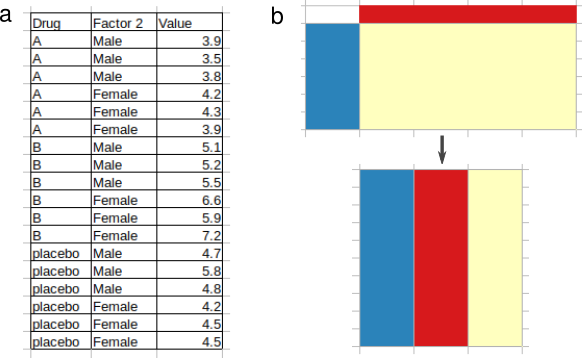Figure 3. Reformatted data in case of replications. (a) The reformatted data from figure 2b. (b) The transformation is visualized by colored blocks where 3 basic factors of a matrix are captured.

In order for the platform to correctly read a data with replications, beside providing the number, users must make sure that the headers are consistently arranged. The headers can be merged (figure 2b) or unmerged (figure 4a and 4b). But in any case, replicates must be placed next to each other.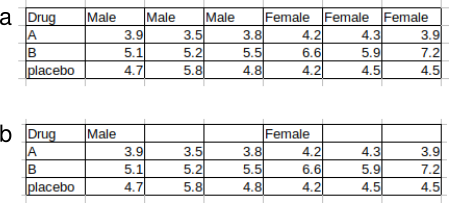Figure 4. Other possible variations of the header (the first row). (a) Headers are repeated accordingly. (b) Headers only label the first replicates.

### Number of replications should be 0 or 1?

You may find it a bit confusing between replication of 0 and 1. If a data has no replication, should we put 1 as the number of replications? In most of the case, the answer is no.

Data transformation will be applied to any data that have a number of replications higher than 0. As discussed, this concept helps users easily take control of the factors during analyses. Figure 5 displays the shape of data remain when the number is 0 but not when the number is 1. Factors are rrug, male’s RBCs, and female’s RBCs in the earlier and drug, gender (Factor 2), and RBCs (Value) in the latter. Though the latter is recommended for data analysis, one might find it is more useful to keep everything original. But keep in mind that the number of replications is different between 0 and 1.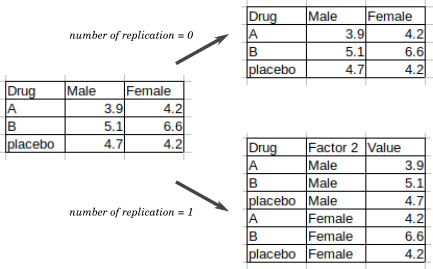Figure 5. Illustration of data transformation when the number of replications is 0 or 1

### It is all about column spanning

In the previous example (see figure 2b), replicates are in the same row but different column. This format is common but not universal. Sometimes, users will have different ways to record their data, as shown in figure 6. Let us assume that the users want to factorize the matrix into drug, gender, and RBCs, or in other words, have their data transformed. What should be the correct number of replications?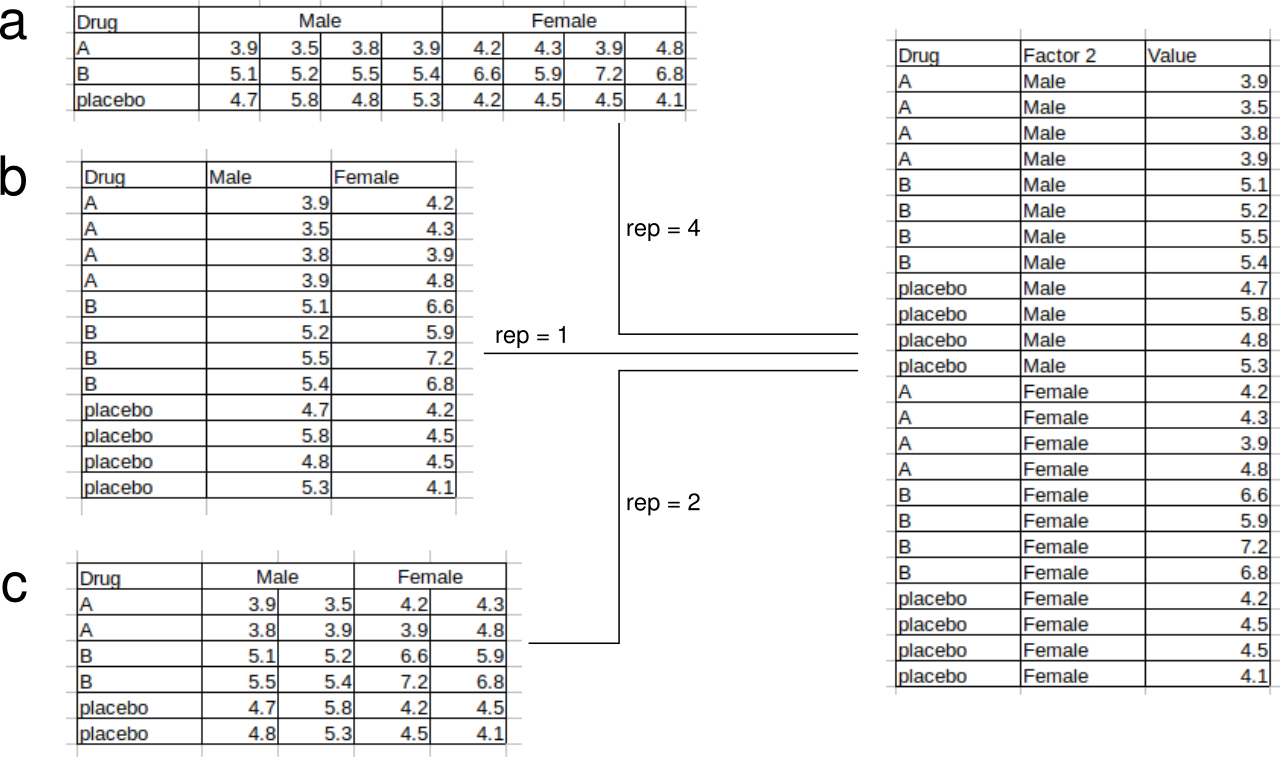Figure 6. Variations of data with replications. (a) Replicates are placed in different columns and the same rows.(b) Replicates are placed in different rows and the same columns. (c) Replicates are placed different columns and rows. “rep” stands for “number of replication”. All examples (a, b, c) show the same data.

In case (a), number of replications should be provided is 3. This is the normal case and we have been discussed it thoroughly. However, in case (b), the correct number is, oddly, 1. Why so? We should keep in mind that BioVinci handles data by columns, where the factor located on the first row becomes a column in transformed version. The number of replications will determine how this factor is stretched to fit with the values which are transformed into a single column as well. Particularly in case (a), the number 3 tells the parser to expand the “male” label into 2 more cells before jumping to the “female” label. Therefore, the number of replications is actually the spanning width of the factor in term of columns. No matter how many rows are spanned, only the number of columns is taken into account. Consequently, in case (c), the correct number of replications is 2, not 4.